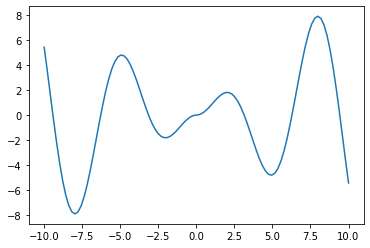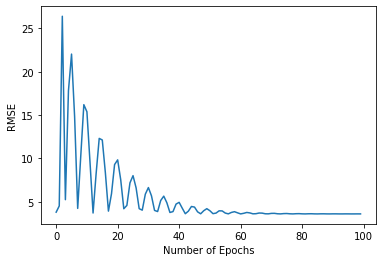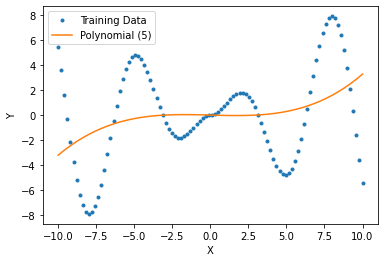# Assignment 1.4: Polynomial Model¶

• Version 1.4: Added division by n_samples in gradient expression. Also inserted the transpose back in to the math expression just before the def polynomial_model line.
• Version 1.3: Changed 'linear model' to 'polynomial model' when referring to what to plot.
• Version 1.2: The mathematical expression just before polynomial_gradient(X, T, W): incorrectly had the transpose of the matrix of powers of X. The transpose has been removed from this most recent version.
• Version 1.1: Added all details, including grading script. Also removed the sentence the steps for defining and plotting X and T for the air quality experiments being different from lecture. It is not different from what was done in lecture.

Replace this line with your name.

In this first assignment, you will write and apply python code that performs gradient descent to fit a polynomial model to the air quality data discussed in the lecture during the first week.

Write code to implement a polynomial that returns the result

$$f(x) = w_0 + w_1 x + w_2 x^2 + \cdots + w_{p-1} x^{p-1}$$

Name this function polynomial_model that is called with two arguments, a column matrix of input values with number of rows equal to the number of samples, and a column matrix of weights with the number of rows equal to the number of powers $p$ to use. Notice that the first term on the right-hand side is actually $w_0 x^0$.

• polynomial_model(X, W):
• Given
• X, an n_samples x 1 numpy array of input samples
• W, an n_powers x 1 numpy array of weight values
• Return
• an n_samples x 1 numpy array of the model's predicted outputs for each sample in X.

Now implement the gradient of the mean-squared-error between the target values in T and the model's output, with respect to the weights, W.

\begin{align*} \nabla_W E &= \nabla_Y E \; \nabla_W Y\\ &= -2 (T - Y) \; \nabla_W Y\\ &= -2 (T - Y) \; [1,\; x,\; x^2,\; x^3,\; \ldots,\; x^{p-1}] \end{align*}

for one sample $x$. With $X$ being a matrix of multiples samples, one per row, we must modify the equation to this. Notice the transpose of the matrix of powers of $X$. The following expression is now divided by n_samples.

$$\nabla_W E = [1,\; X,\; X^2,\; X^3,\; \ldots,\; X^{p-1}]^T \;(-2)\; (T - Y) \;/\; \text{n_samples}$$

\;

• polynomial_gradient(X, T, W):
• Given
• X, an n_samples x 1 numpy array of input samples
• T, an n_samples x 1 numpy array of correct outputs (targets) for each input sample
• W, an n_powers x 1 numpy array of weight values
• Return
• an n_powers x 1 numpy array of the gradient of the mean squared error with respect to each weight. (Same shape is W.)

Download the air quality data and prepare the X and T matrices as shown in the following code cells. Plot CO(GT) air quality (on the y axis) versus the hour of the day (on the x axis) to verify you have prepared the data correctly.

Use the gradient_descent_adam function defined in the lecture notes to find the best weights for the polynomial model, as illustrated in lecture. Plot the RMSE versus iterations, plot the weights versus the number of steps, and plot the air quality versus hour of the day again and superimpose the polynomial model on the same graph.

## Simple Test of your Code¶

Let's copy and paste two of the functions used in lecture for use here.

In :
import numpy as np
import matplotlib.pyplot as plt
import pandas

In :
def gradient_descent_adam(model_f, gradient_f, rmse_f, X, T, W, rho, nSteps):
# Commonly used parameter values
alpha = rho
beta1 = 0.9
beta2 = 0.999
epsilon = 1e-8
m = 0
v = 0

error_sequence = []
W_sequence = []
for step in range(nSteps):
error_sequence.append(rmse_f(model_f, X, T, W))
W_sequence.append(W.flatten())

m = beta1 * m + (1 - beta1) * g
v = beta2 * v + (1 - beta2) * g * g
mhat = m / (1 - beta1 ** (step+1))
vhat = v / (1 - beta2 ** (step+1))
W -= alpha * mhat / (np.sqrt(vhat) + epsilon)

return W, error_sequence, W_sequence

def rmse(model, X, T, W):
return np.sqrt(np.mean(  (T - model(X, W)) **2 )  )

In :
X = np.linspace(-10, 10, 100).reshape(-1, 1)
T = np.sin(X) * np.abs(X)
plt.plot(X, T);

Out:
[<matplotlib.lines.Line2D at 0x7f4c3c25b190>]In :
n_powers = 5
W = np.zeros((n_powers, 1))  # Initial weights

rho = 0.01  # learning rate
n_steps = 100  # number of updates to W

rmse,
X, T, W,
rho, n_steps)

In :
plt.plot(error_sequence)
plt.xlabel('Number of Epochs')
plt.ylabel('RMSE');In :
plt.plot(X, T, '.', label='Training Data')
plt.plot(X, polynomial_model(X, W), label=f'Polynomial ({n_powers})')
plt.xlabel('X')
plt.ylabel('Y')
plt.legend();# Air Quality Data¶

Download the air quality data and prepare the X and T matrices as shown in the following code cells. When done correctly, X and T should both have shape (827, 1). Plot CO(GT) air quality (on the y axis) versus the hour of the day (on the x axis) to verify you have prepared the data correctly.

Use the gradient_descent_adam function defined in the lecture notes to find the best weights for the polynomial model, as illustrated in lecture. Plot the RMSE versus iterations, plot the weights versus the number of steps, and plot the air quality versus hour of the day again and superimpose the polynomial model on the same graph.

Now apply the Adam optimization function to fit a polynomial to this data. Try several different values of n_powers and n_steps. Plot the results and describe what you see.

Your notebook will be run and graded automatically. Test this grading process by first downloading A1grader.tar (to be provided soon) and extract A1grader.py from it. Run the code in the following cell to demonstrate an example grading session. You should see a perfect execution score of 60/60 if your functions are defined correctly. The remaining 40 points will be based on other testing and the results you obtain and your discussions.

A different, but similar, grading script will be used to grade your checked-in notebook. It will include additional tests. You should design and perform additional tests on all of your functions to be sure they run correctly before checking in your notebook.

For the grading script to run correctly, you must first name this notebook as 'Lastname-A1.ipynb' with 'Lastname' being your last name, and then save this notebook.

In :
%run -i A1grader.py

======================= Code Execution =======================

Extracting python code from notebook named 'Anderson-A1.ipynb' and storing in notebookcode.py
Removing all statements that are not function or class defs or import statements.

Testing
X = np.array([1, -2, 3, -4, 5, -8, 9, -10]).reshape((-1, 1))
W = np.ones((4, 1))
Y = polynomial_model(X, W)

--- 20/20 points. Returned correct values.

Testing
X = np.array([1, -2, 3, -4, 5, -8, 9, -10]).reshape((-1, 1))
W = np.ones((4, 1))
Y = polynomial_model(X, W)
T = np.array([[   4.2],
[  -4.8],
[  40.2],
[ -50.8],
[ 156.2],
[-454.8],
[ 820.2],
[-908.8]])

--- 20/20 points. Returned correct values.

Testing
X = np.array([1, 2, 3, 4, 5, 8, 9, 11]).reshape((-1, 1))
T = (X - 5) * 0.05 + 0.002 * (X - 8)**2
W = np.zeros((5, 1))
rho = 0.01
n_steps = 100
X, T, W, rho, n_steps)

--- 20/20 points. Returned correct values.

======================================================================
A1 Execution Grade is 60 / 60
======================================================================

__ / 10 Reading in air quality data and plotting it correctly.

__ / 10 points. Applying the Adam optimizer to the air quality data using your polynomial model and gradient correctly.

__ / 10 points.  Plotting the resulting error curve in one graph and plotting the model predictions on top of data
correctly.  Also describe what you observe in these two graphs with at least five total sentences.

__ / 10 points. Show and describe results for three different values of n_powers and also for three different values of n_steps,
Describe what you see with at least eight sentences.

======================================================================
A1 Results and Discussion Grade is ___ / 40
======================================================================

======================================================================
A1 FINAL GRADE is  _  / 100
======================================================================


# Check-In¶

Do not include this section in your notebook.

Name your notebook Lastname-A1.ipynb. So, for me it would be Anderson-A1.ipynb. Submit the file using the Assignment 1 link on Canvas.